# Alkynes – Organic Chemistry – list of solved problems

Go to the page with the solutions to the problems.

## Alkynes – list of problems

### Problem 1)

Complete the following reaction:

pent-2-ino + H2 (Lindlar’s catalyst) →

### Problem 2)

Predict the result of the following transformation:

hex-3-ino + H2 (Na / NH3) →

### Problem 3)

State the final product obtained by addition of one mole of HCl to but-2-ino.

### Problem 4)

In the above case, what will be the result if an excess of HCl is used?

### Problem 5)

Complete the following reaction, justifying the result.

3,3-dimethylbut-1-ino + 2 HBr →

### Problem 6)

What will be the result of the addition of one mole of HBr over 3,3-dimethylbut-1-ino in the presence of peroxides? Describe the mechanism that takes place in such a transformation.

### Problem 7)

Describe what will be the result of the addition of one mole and two moles of Cl2 independently over hex-3-ino in dichloromethane.

### Problem 8)

The acid-catalyzed addition of water over hex-3-ino leads to the formation of a carbonyl compound. Justify this result and compare it with the product obtained under the same conditions with hex-3-ene.

### Problem 9)

The addition reaction of water on but-1-ino is carried out by H2SO4/HgSO4 catalysis. Propose a mechanism for this transformation.

### Problem 10)

Indicate what will be the result of the reaction of borane on but-1-ino followed by treatment with hydrogen peroxide in basic medium.

### Problem 11)

Predict the major product that would be obtained from but-1-ino in each of the following transformations, indicating if applicable, the regio- and stereo-chemistry of the process, as well as the reagents and reaction conditions:

• a) addition of 1 mol of halogen
• b) hydroboration/oxidation
• c) catalytic hydrogenation
• d) reduction with metals
• e) oxidation with permanganate

### Problem 12)

Repeat the same previous problem 11) starting from but-2-ino, proposing a mechanism for process c).

### Problem 13)

Complete the following scheme (A-H):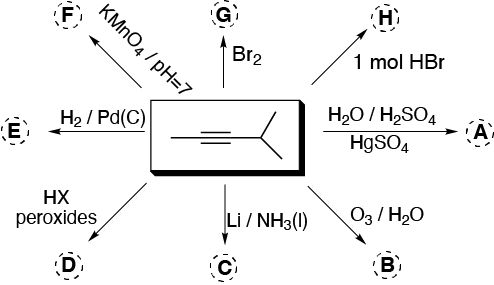### Problem 14)

Starting from alkynes (I-III), choose the most appropriate reaction conditions to obtain the products (a-h) given below: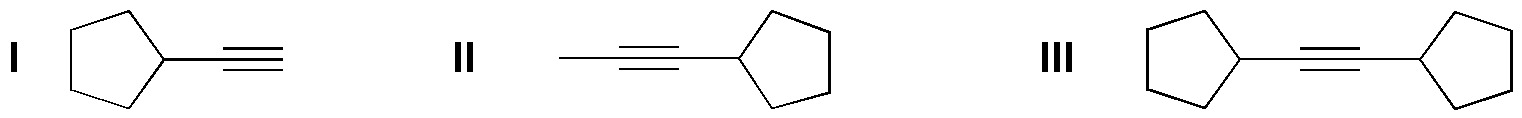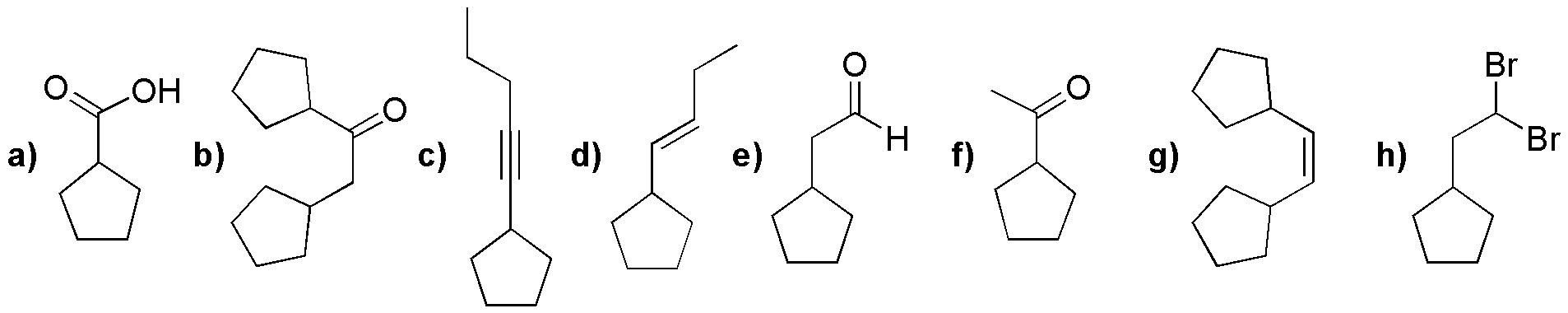### Problem 15)

The reactions given in the following scheme are incorrect. Explain what the error consists of, and what would be the product obtained.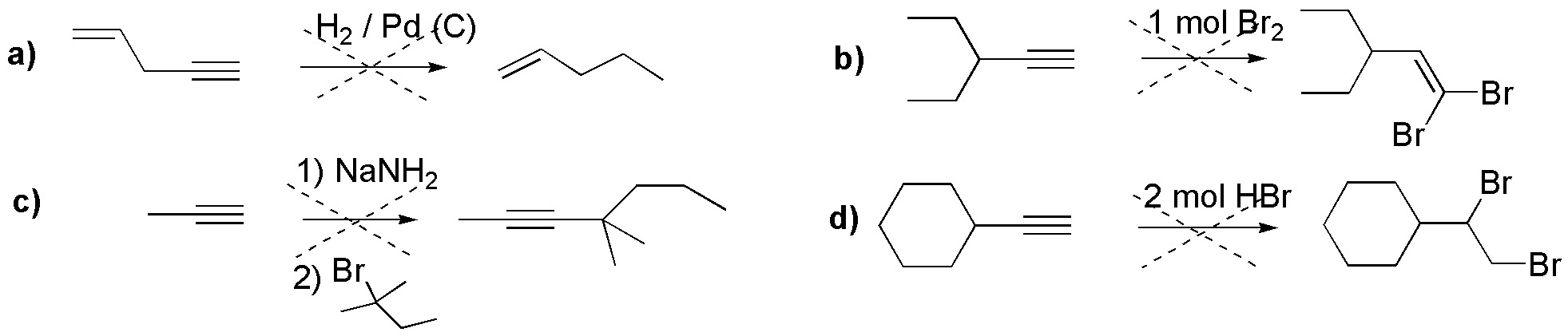### Problem 16)

Indicate, explaining the possible mechanism, the result of the addition of one mole of bromine in water to 1-butene.

### Problem 17)

Complete the following scheme (A-I):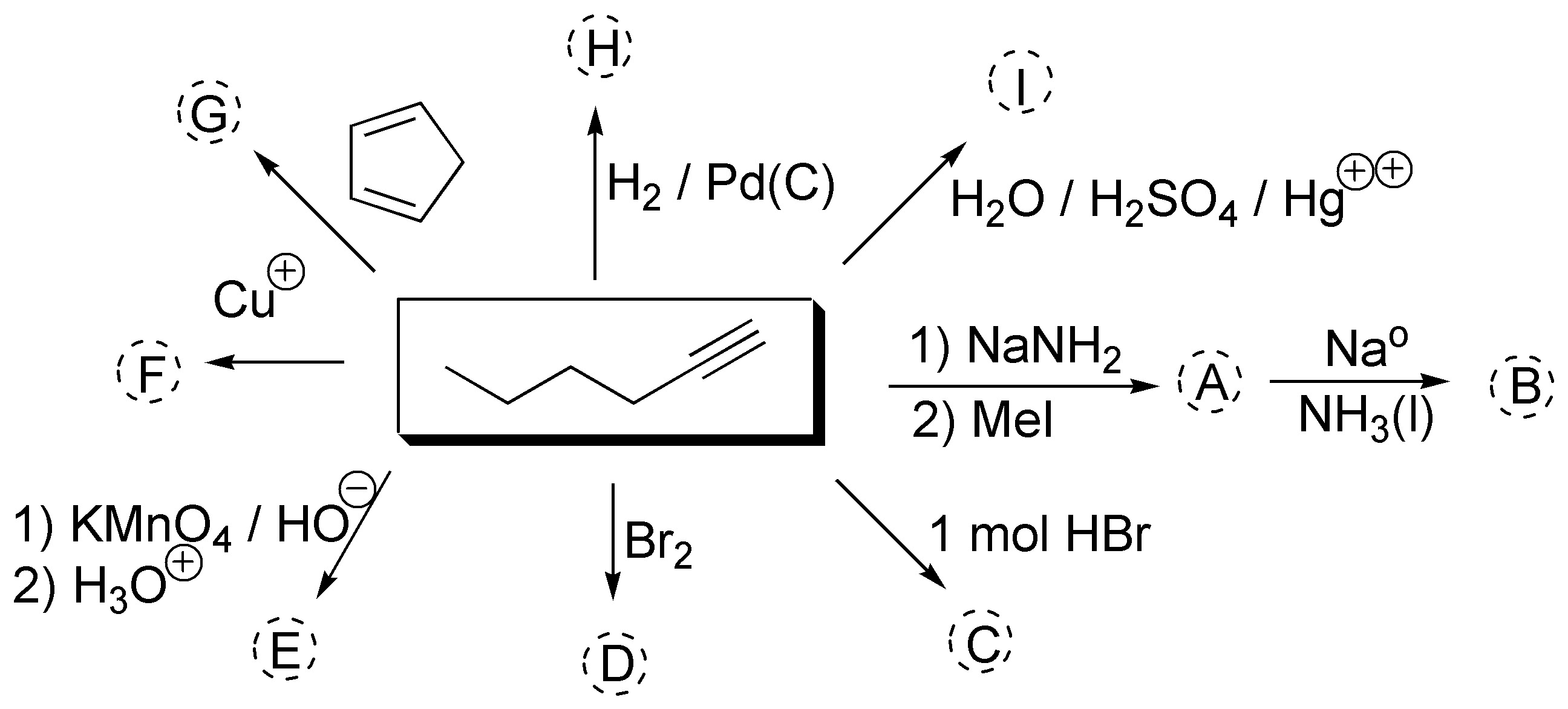### Problem 18)

Complete the following reactions (a-f) indicating the appropriate reagent (A-F) in each case.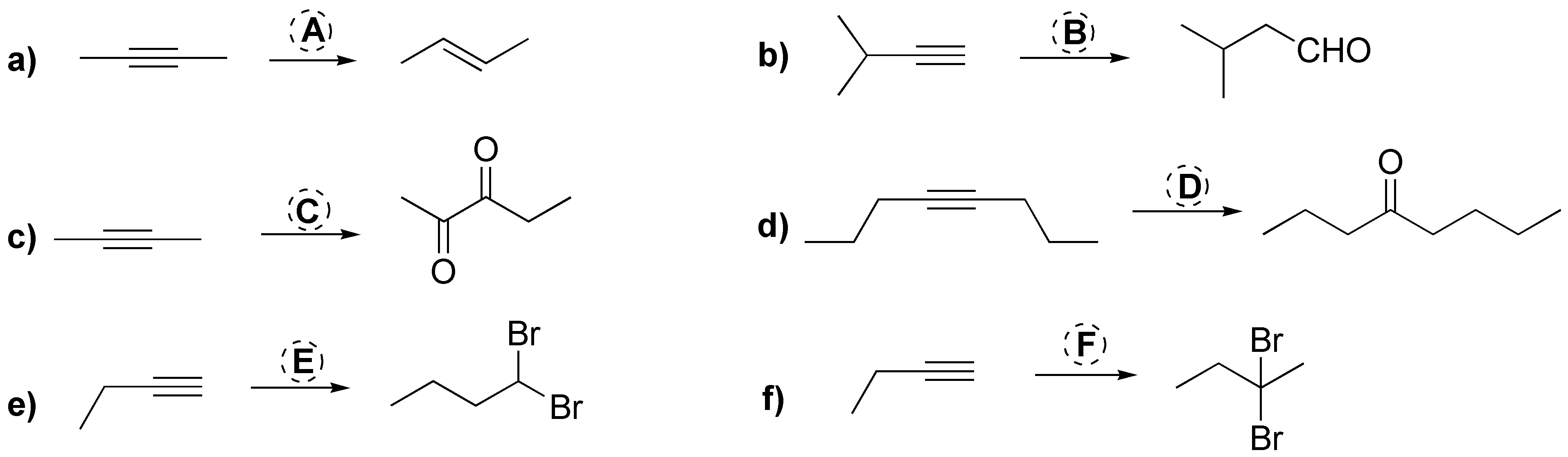### Problem 19)

Suggest an explanation for the increasing order of acidity alkyne > alkene > alkane.

### Problem 20)

Identify the fragments obtained by ozonolysis of the following alkynes: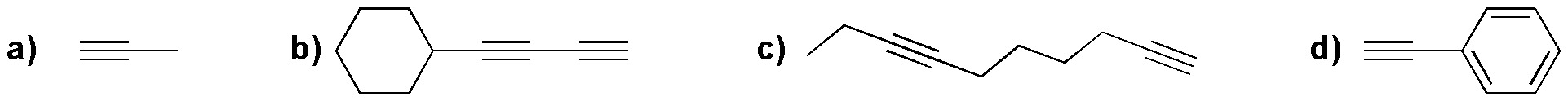### Problem 21)

Complete the following reactions: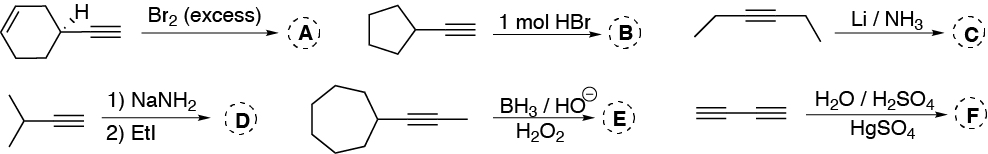### Problem 22)

Of the following bases (a-e), choose the one most suitable for the preparation of acetylides:

• a) NaOH
• b) NaH
• c) NaMeO
• d) NaNH2
• e) NaHCO3

### Problem 23)

Choose, from the following list, the most suitable reagent for the transformations (A-F) given:

• 1 mol Br2
• 2 moles Br2
• 2 moles HBr
• BH3 / HO / H2O2
• H2 / Pt
• H2 / Lindlar catalyst
• H2O / H2SO4/ HgSO4
• HBr / peroxides
• KMnO4 / HO / H3O+
• KMnO4 / pH=7
• Li / NH3(l)
• O3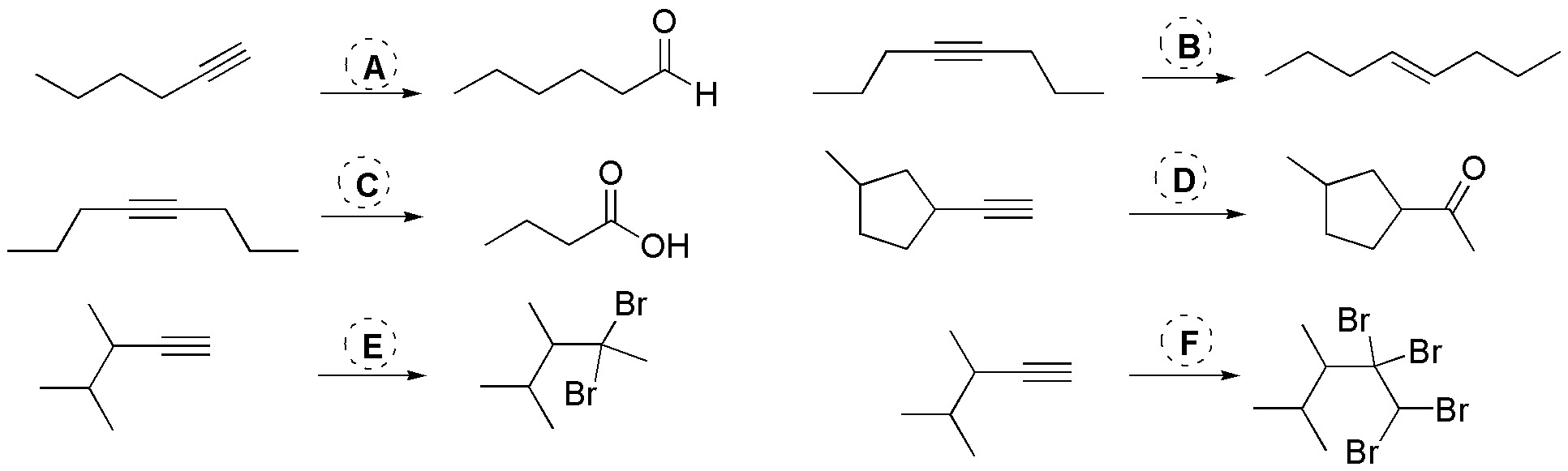### Problem 24)

Indicate the reaction conditions and reagents required to, from acetylene, obtain:

• a) pent-2-ino
• b) (E)-pent-2-ene
• c) (Z)-pent-2-ene
• d) pentane
• e) acetone
• f) propanal

### Problem 25)

Devise a synthesis to obtain the following compound from ethene (acetylene).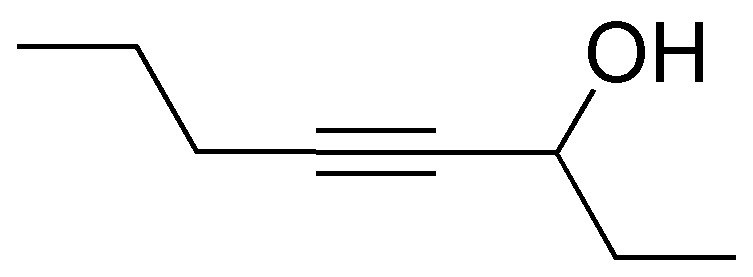### Problem 26)

What product(s) or will be obtained in the acid-catalyzed hydration and mercuric salts of the following alkynes?

• a) ethyne
• b) propyne
• c) 1-butene
• d) 2-butene
• e) 2-methylhex-3-ino

### Problem 27)

Indicate what would be the products obtained from propino by treatment with the following reagents:

• a) D2 / Lindlar cat.
• b) Na / liquid ND3
• c) 1 equivalent of HI
• d) 2 equivalents of ICl
• e) 2 equivalents of HI
• f) 2 equivalents of bromine
• g) H2O / H2SO4 / HgSO4
• h) Dicyclohexylborane and subsequent treatment with H2O2 in a basic medium.
Shares